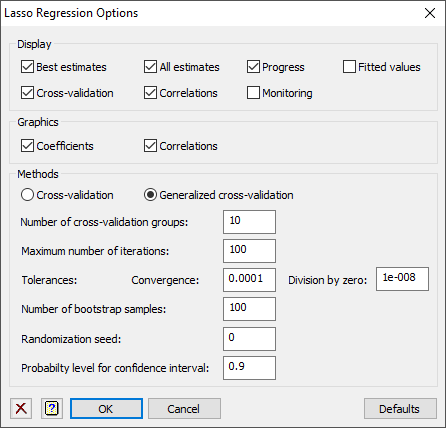1. Home
2. Lasso Regression Options

# Lasso Regression Options

Use this to select different options to be used in a Lasso regression.## Display

Specifies which items of output are to be displayed in the Output window.

 Best estimates The lasso estimates for the optimal λ All estimates For each value of λ, the lasso coefficients and their standard errors on the standardized and original scales Progress Shows the progress of the k-fold cross-validation Cross-validation The cross-validation results, with optimal λ value Correlation The correlations between the explanatory variables in the model formula

## Graphics

This controls what graphical plots are displayed from the analysis:

 Coefficients Plots the standardized coefficient estimates against the shrinkage factor, and correlations Correlations Uses the DCORRELATION procedure to produce a graphical representation of the correlation matrix for elements in the model formula

## Methods

This controls how the optimal value of λ is selected.

 Cross-validation Use k-fold cross-validation where the prediction error is calculated using the mean squared error Generalized cross-validation Use the generalized cross-validation, as specified by Tibshirani (1996) – see RLASSO for full reference

## Number of cross-validation groups

For the cross-validation, this gives the number of groups the data will be allocated to. Each group is then left out of the analysis and predicted from the remaining groups. On each run, the units are randomly assigned to groups using the randomization seed.

## Convergence tolerance

Specifies convergence criterion for the iterative least-squares. This is a small number that controls when the model fitting process stops.

## Division by zero tolerance

Specifies a small adjustment to avoid division by zero in the penalty term.

## Maximum number of iterations

This option specifies the maximum number of iterations that can be used for the iterative process of model fitting, default 100.

## Randomization seed

Specifies the seed for the random number generator used to make the cross-validation groups; default 0 continues from the previous generation or (if none) initializes the seed automatically.